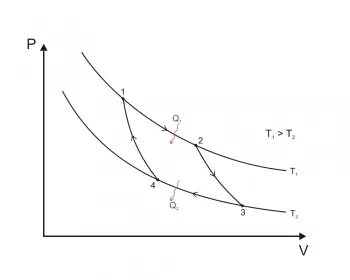# Laws of ThermodynamicsIn the various theoretical descriptions of thermodynamics, these laws can be expressed in apparently different ways. However, the most prominent formulations are as follows:

## Zeroth Law of Thermodynamics

This principle is called the thermodynamic equilibrium. If two systems A and B are in thermodynamic equilibrium, and B is in thermodynamic equilibrium with a third system C, then A and C are in turn in thermodynamic equilibrium.

### What Does It Mean That a Process Is in Thermodynamic Equilibrium?

The thermodynamic equilibrium of a thermodynamic system is defined as the condition of the system in which the empirical variables used to define a state of the system have reached an equilibrium point. Being in balance, they do not vary over time.

There is no transfer of energy.

## The First Law of Thermodynamics

The first low states that "energy of an isolated system is neither created nor destroyed, it remains constant". Energy can be converted from one form to another but not created nor destroyed.

You can pass from one form of energy to another, but energy is neither created nor disappears. For example, in a heat engine, the thermal energy of combustion energy can be converted into kinetic energy or into electrical energy.

This law allows defining heat as the amount of necessary energy that the system must exchange to compensate for the differences between work done by the system and internal energy.

## The Second Law of Thermodynamics

The second law of thermodynamics regulates the direction in which thermodynamic processes have to be carried out. In some cases, it is not possible to carry them out in the opposite direction.

This law allows us to define entropy. The variation in the amount of entropy of an isolated thermodynamic system must always be greater than or equal to zero. It is only when it is equal to zero that the process is reversible.

## The Third Law of Thermodynamics

The third of the laws of thermodynamics states that it is impossible to reach a temperature equal to absolute zero through a finite number of physical processes.

When the temperature approaches absolute zero, the entropy of any system approaches zero.

Author:

Published: August 28, 2018
Last review: August 27, 2020